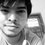# Introduction

Crypt-arithmetic is the science and art of creating and solving Cryptarithm which is a type of mathematical game consisting of a mathematical equation among unknown numbers, whose digits are replaced or represented by letters. The goal is to identify the value of each other for proper functioning of the underlying equations which are typically basic operations of arithmetic like addition, subtraction, multiplication and division.

The invention of crypt-arithmetic has not yet been ascribed to some specific country, place or time. The first use of the crypt-arithmetic problems or puzzles is also still unknown as its history is often debated on. Sam Loyd is often said to be the inventor of them. The term "cryptarithmie" was coined by puzzlist Minos aka Simon Vatriquant in the 1931 May issue of Sphinx, a belgian magazine of recreational mathematics. Before that in 1924, July Henry Dudeney published a classic example in the Strand Magazine associating with the story of a kidnapper’s ransom demand. Through out the span of Sphinx, it has offered a handful of good crypt-arithmetic problems.

Crypt-arithmetic problems are often broadly classified into four categories : $Alphametic$ - Where letters of alphabet replaces the decimal digits. $Digimetic$ - Where digits are used to represent other digits. $Skeletal division$ - A long division where all or most digits are replaced by asterisks. $Reverse crypt-arithmetic$ - Where a formula is written and the solution is the corresponding cryptarithm whose solution is the formula given.

This set delves into all types of the above-mentioned four categories distinctly. However the set doesn’t dig deep into the detailed solutions of them. The readers are encouraged to solve them by hand themselves. The use of modular arithmetic sometimes may help you to solve them.Crypt-arithmetic not only finds its application in recreational mathematics and puzzles but in computer science, they provide good examples to illustrate Brute Force method and algorithms that generate all permutations of m choices from n possibilities. They also provide good examples for backtracking paradigm of algorithm design.Note by Sayan Chaudhuri
3 years, 6 months ago

This discussion board is a place to discuss our Daily Challenges and the math and science related to those challenges. Explanations are more than just a solution — they should explain the steps and thinking strategies that you used to obtain the solution. Comments should further the discussion of math and science.

When posting on Brilliant:

• Use the emojis to react to an explanation, whether you're congratulating a job well done , or just really confused .
• Ask specific questions about the challenge or the steps in somebody's explanation. Well-posed questions can add a lot to the discussion, but posting "I don't understand!" doesn't help anyone.
• Try to contribute something new to the discussion, whether it is an extension, generalization or other idea related to the challenge.

MarkdownAppears as
*italics* or _italics_ italics
**bold** or __bold__ bold
- bulleted- list
• bulleted
• list
1. numbered2. list
1. numbered
2. list
Note: you must add a full line of space before and after lists for them to show up correctly
paragraph 1paragraph 2

paragraph 1

paragraph 2

[example link](https://brilliant.org)example link
> This is a quote
This is a quote
    # I indented these lines
# 4 spaces, and now they show
# up as a code block.

print "hello world"
# I indented these lines
# 4 spaces, and now they show
# up as a code block.

print "hello world"
MathAppears as
Remember to wrap math in $$ ... $$ or $ ... $ to ensure proper formatting.
2 \times 3 $2 \times 3$
2^{34} $2^{34}$
a_{i-1} $a_{i-1}$
\frac{2}{3} $\frac{2}{3}$
\sqrt{2} $\sqrt{2}$
\sum_{i=1}^3 $\sum_{i=1}^3$
\sin \theta $\sin \theta$
\boxed{123} $\boxed{123}$